Скачать презентацию 1 A linear equation whose graph goes through

d1a6ff400e0d1a29b6dda1f6489d9f0c.ppt

• Количество слайдов: 34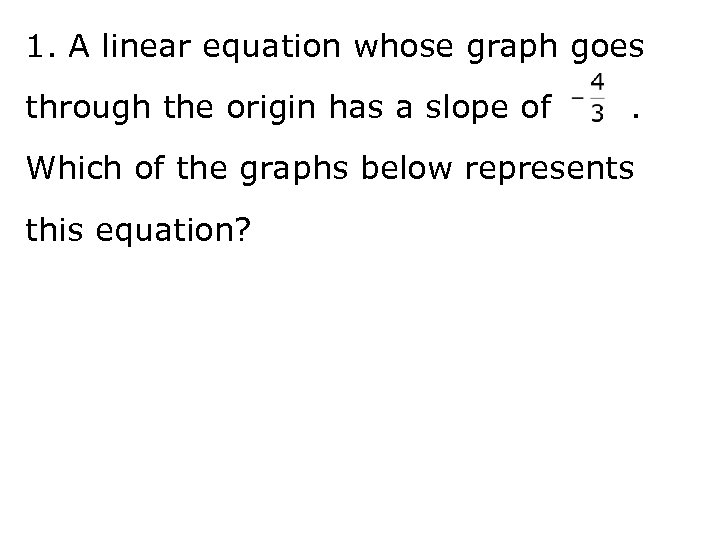1. A linear equation whose graph goes through the origin has a slope of . Which of the graphs below represents this equation?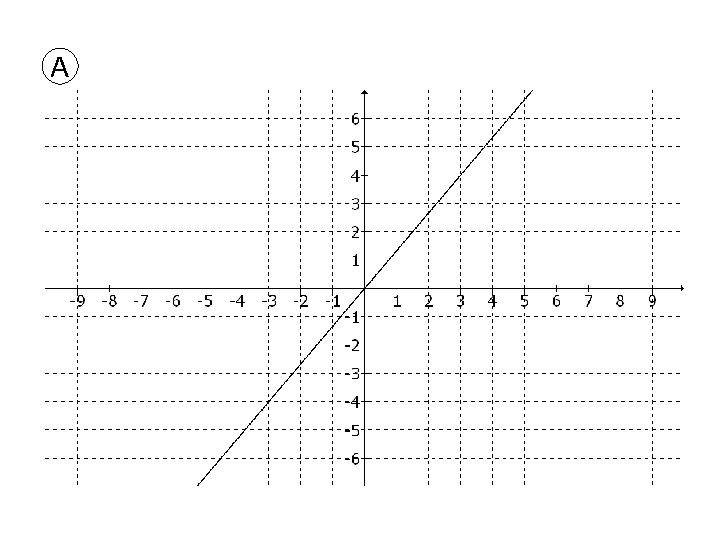A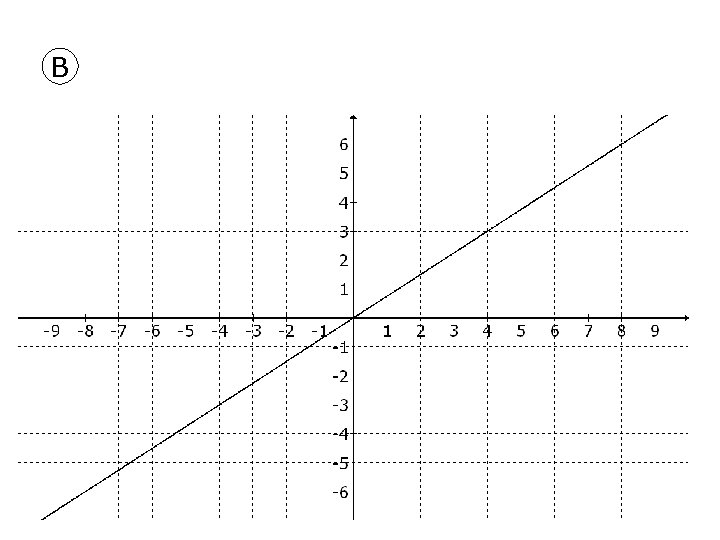B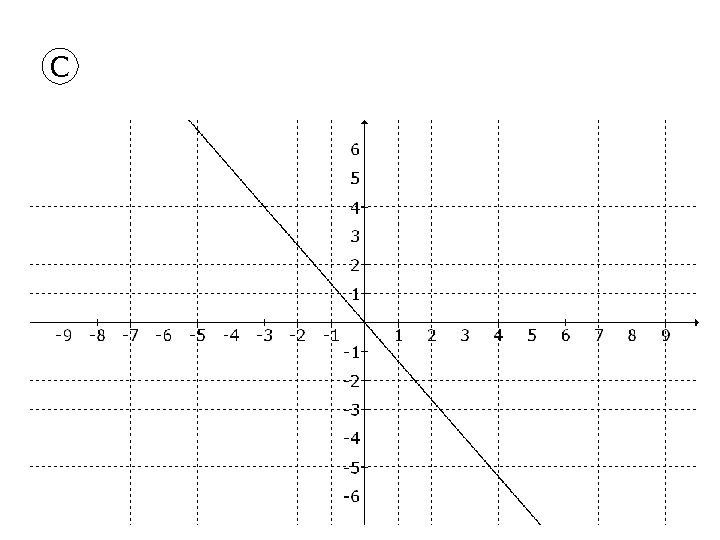C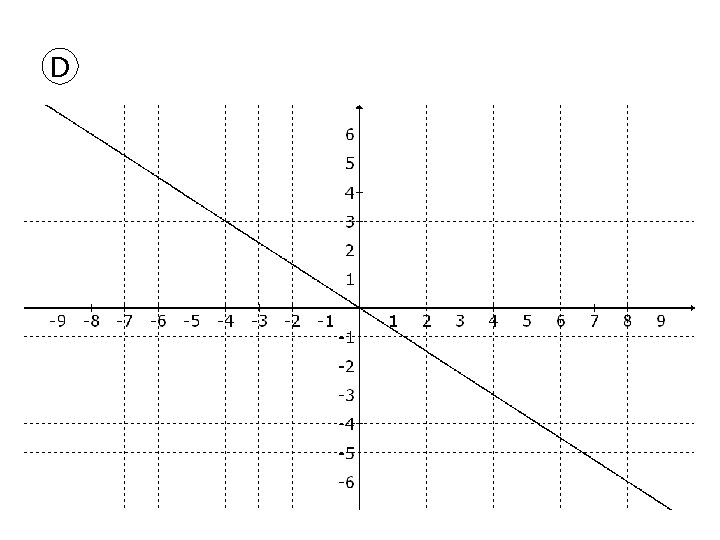D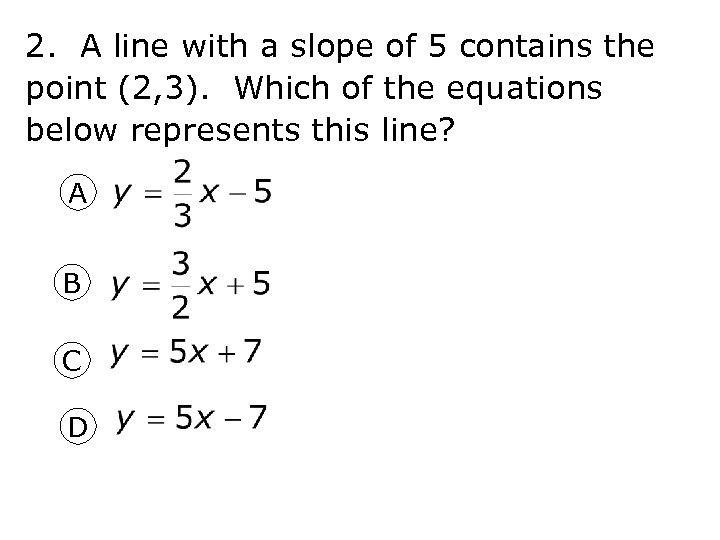2. A line with a slope of 5 contains the point (2, 3). Which of the equations below represents this line? A B C D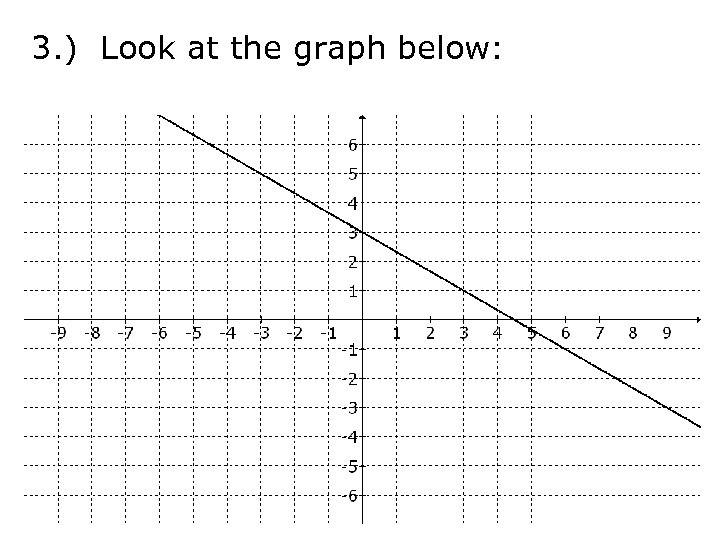3. ) Look at the graph below: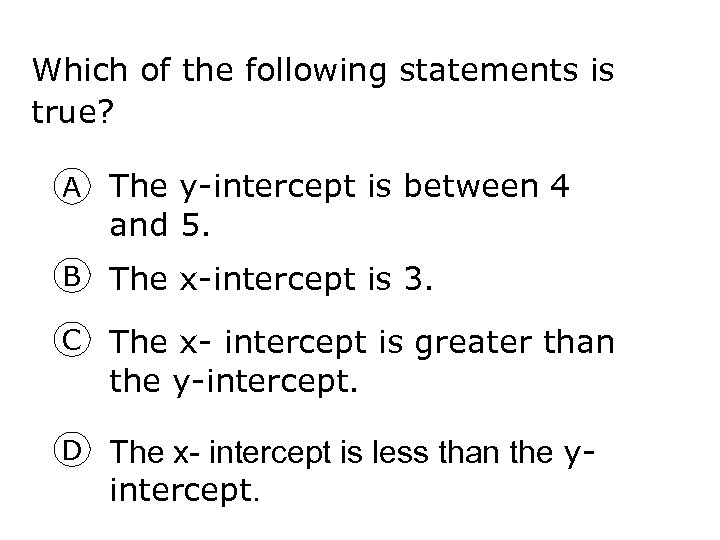Which of the following statements is true? A The y-intercept is between 4 and 5. B The x-intercept is 3. C The x- intercept is greater than the y-intercept. D The x- intercept is less than the y- intercept.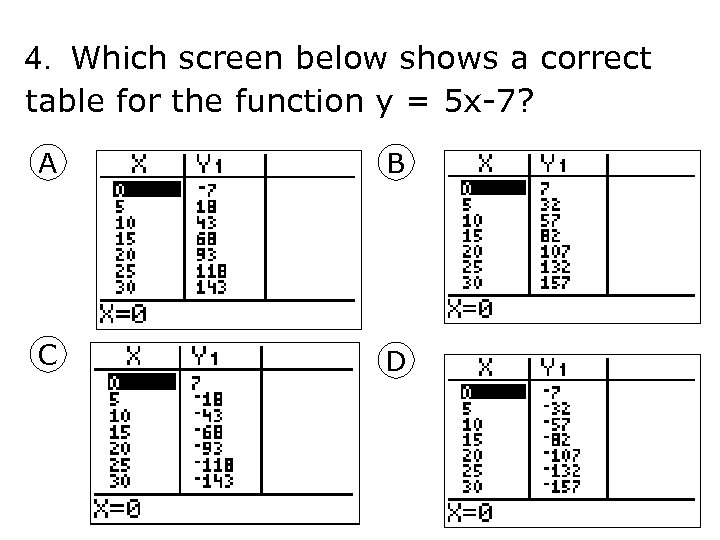4. Which screen below shows a correct table for the function y = 5 x-7? A B C D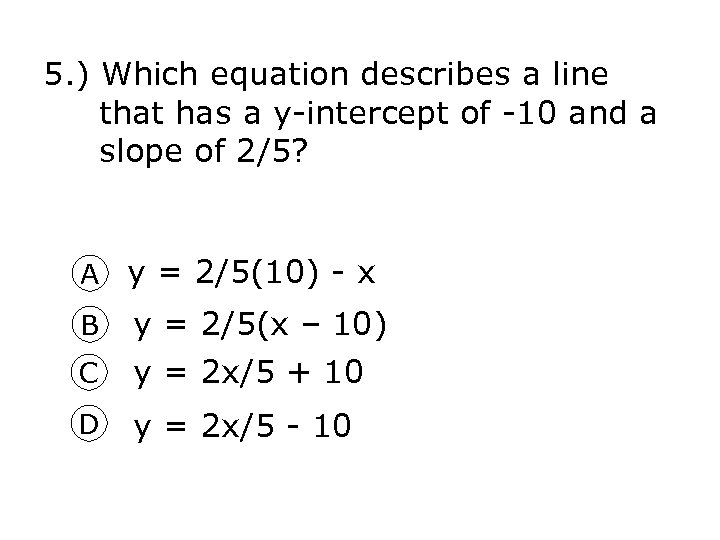5. ) Which equation describes a line that has a y-intercept of -10 and a slope of 2/5? A y = 2/5(10) - x C y = 2/5(x – 10) y = 2 x/5 + 10 D y = 2 x/5 - 10 B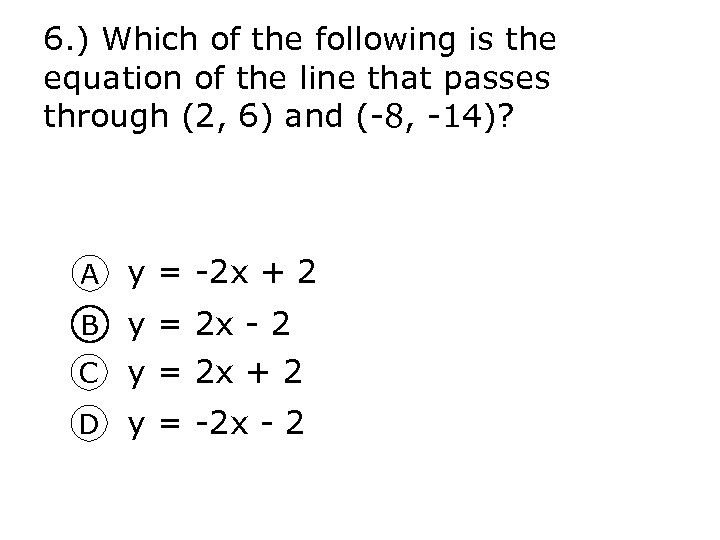6. ) Which of the following is the equation of the line that passes through (2, 6) and (-8, -14)? A y = -2 x + 2 B y = 2 x - 2 C y = 2 x + 2 D y = -2 x - 2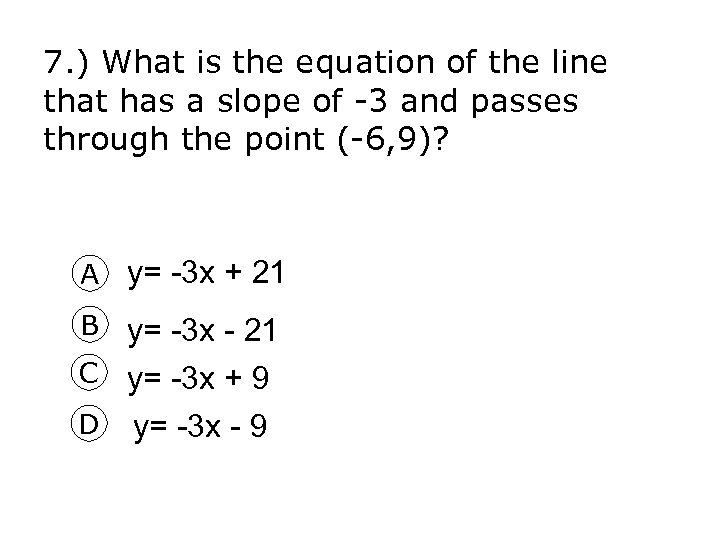7. ) What is the equation of the line that has a slope of -3 and passes through the point (-6, 9)? A y= -3 x + 21 B y= -3 x - 21 C y= -3 x + 9 D y= -3 x - 9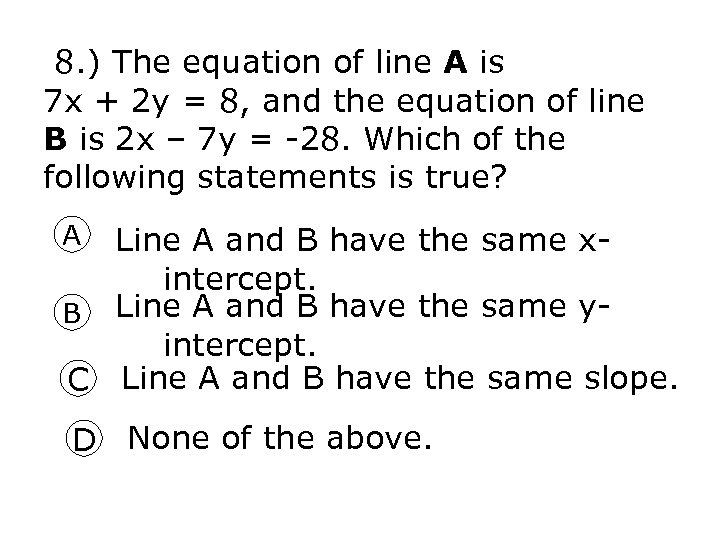8. ) The equation of line A is 7 x + 2 y = 8, and the equation of line B is 2 x – 7 y = -28. Which of the following statements is true? A Line A and B have the same x- intercept. B Line A and B have the same yintercept. C Line A and B have the same slope. D None of the above.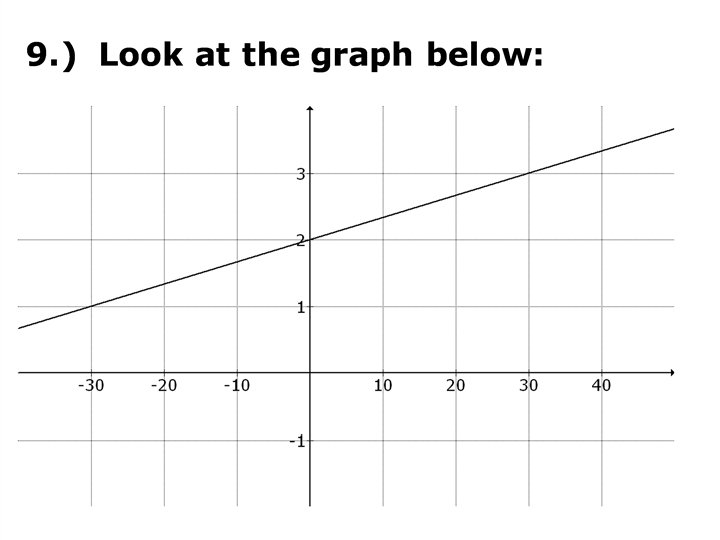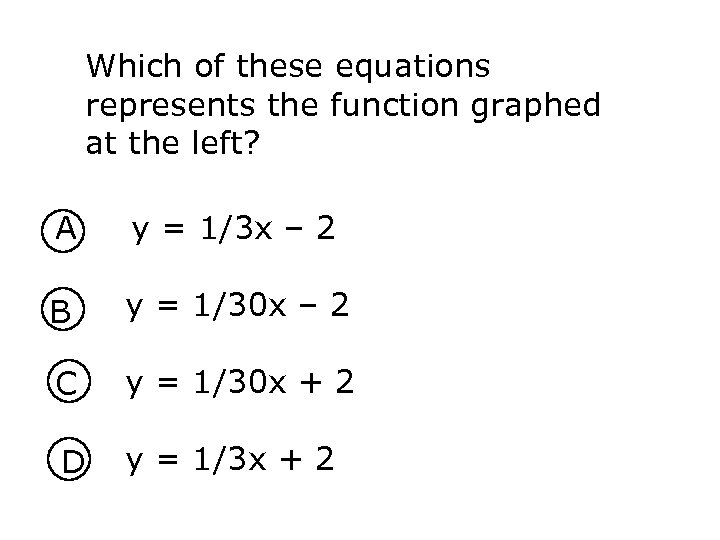Which of these equations represents the function graphed at the left? A y = 1/3 x – 2 B y = 1/30 x – 2 C y = 1/30 x + 2 D y = 1/3 x + 2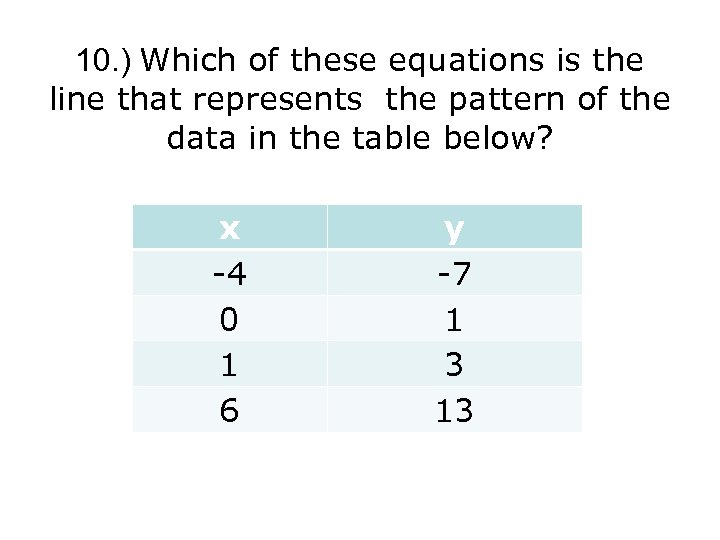10. ) Which of these equations is the line that represents the pattern of the data in the table below? x -4 0 1 6 y -7 1 3 13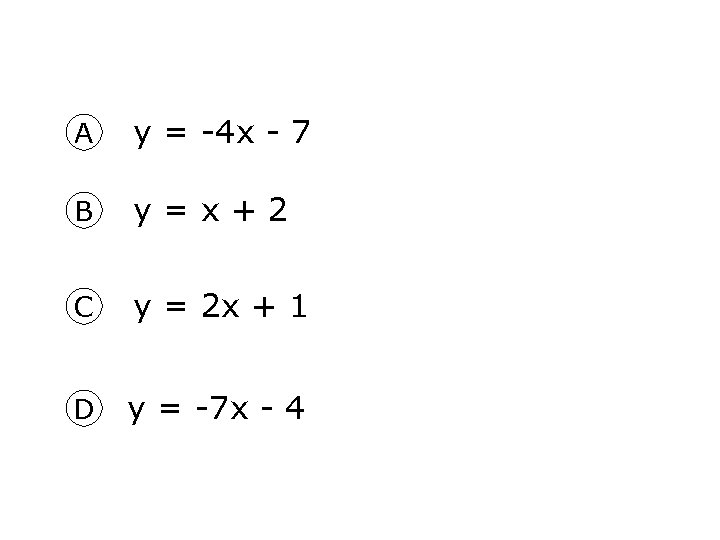A y = -4 x - 7 B y = x + 2 C y = 2 x + 1 D y = -7 x - 4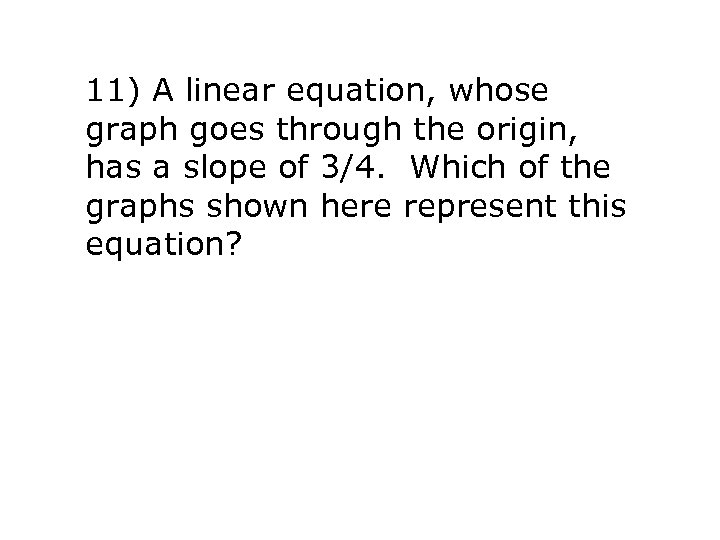11) A linear equation, whose graph goes through the origin, has a slope of 3/4. Which of the graphs shown here represent this equation?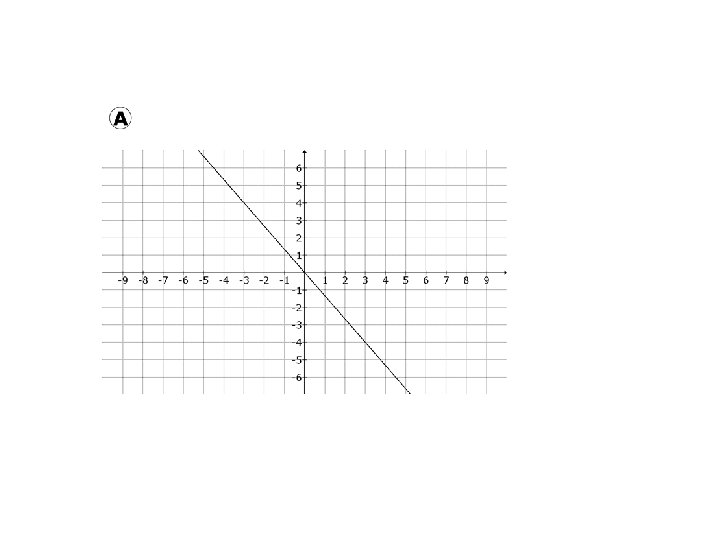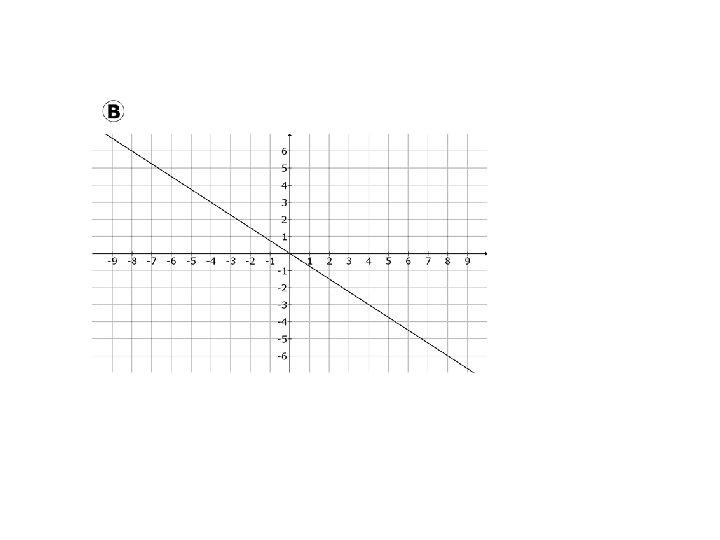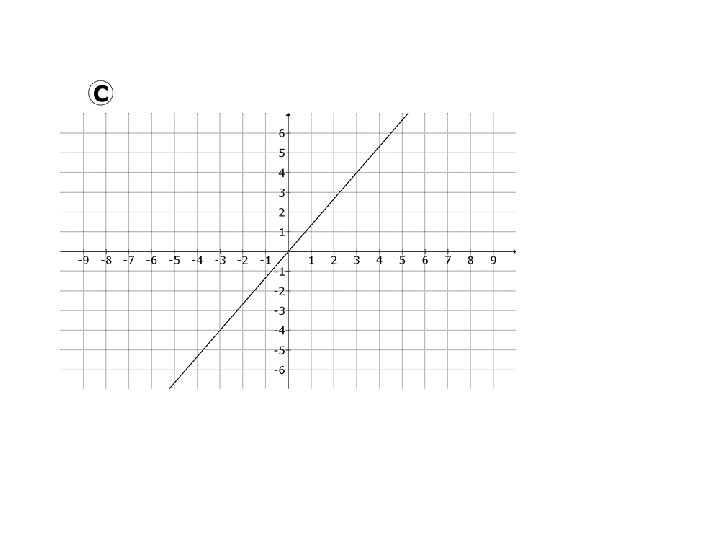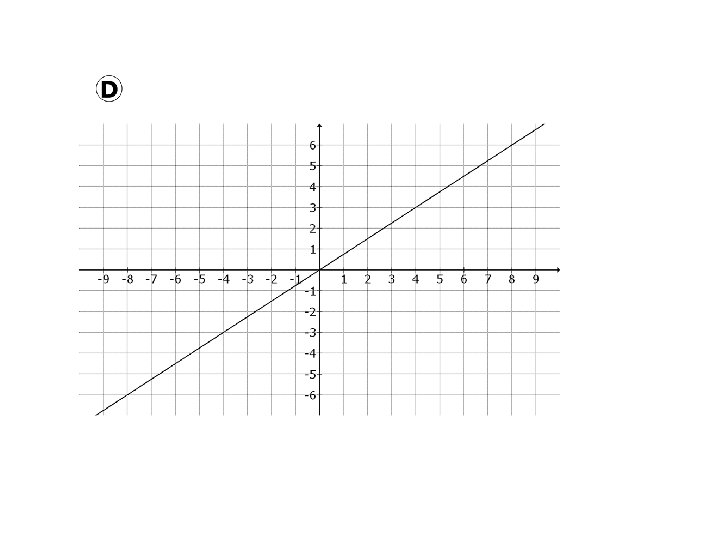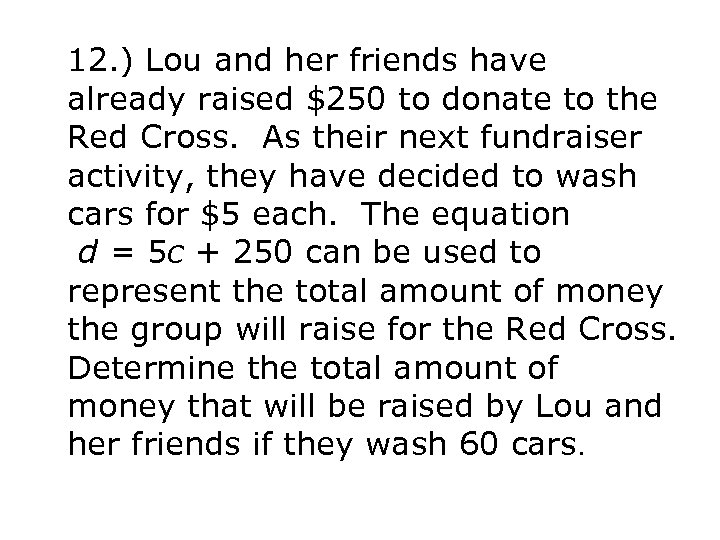12. ) Lou and her friends have already raised \$250 to donate to the Red Cross. As their next fundraiser activity, they have decided to wash cars for \$5 each. The equation d = 5 c + 250 can be used to represent the total amount of money the group will raise for the Red Cross. Determine the total amount of money that will be raised by Lou and her friends if they wash 60 cars.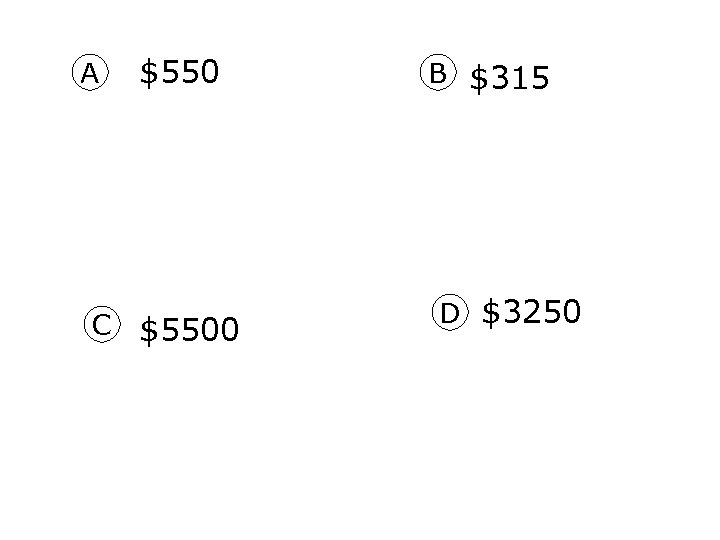A \$550 C \$5500 B \$315 D \$3250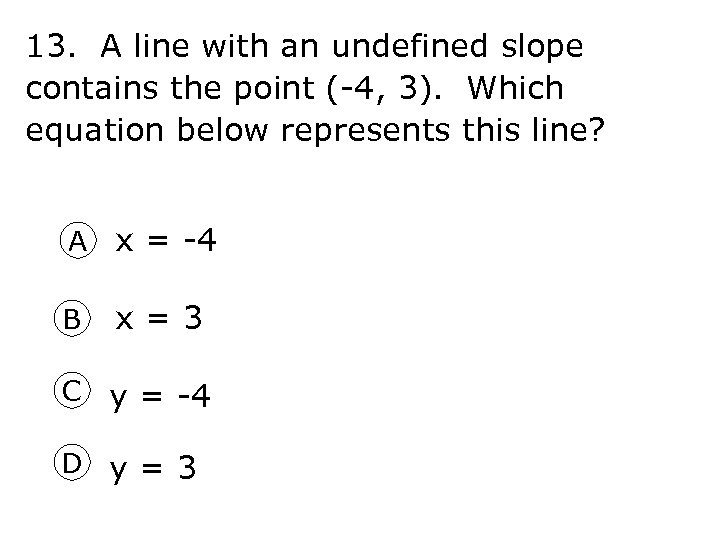13. A line with an undefined slope contains the point (-4, 3). Which equation below represents this line? A x = -4 B x = 3 C y = -4 D y = 3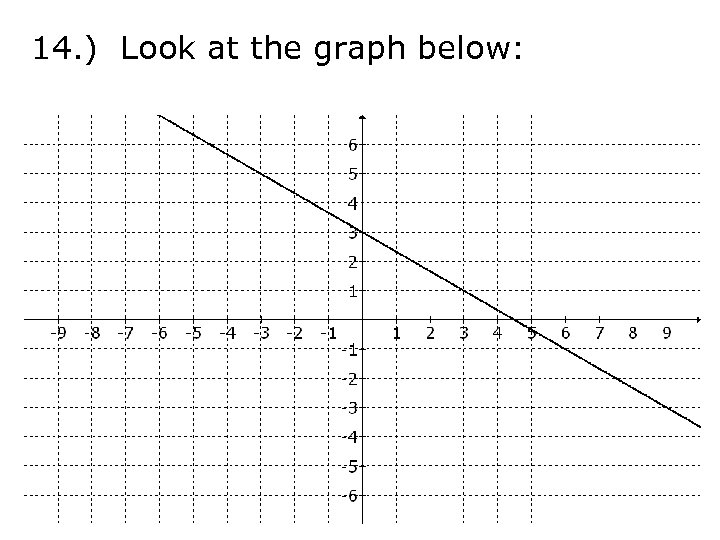14. ) Look at the graph below: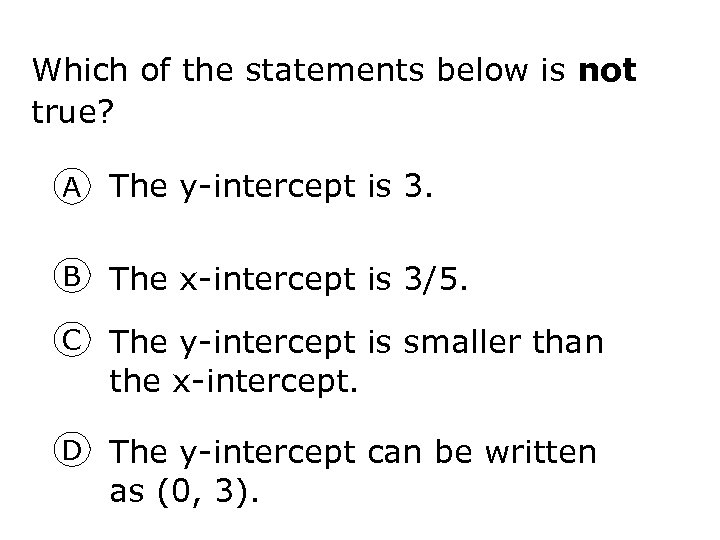Which of the statements below is not true? A The y-intercept is 3. B The x-intercept is 3/5. C The y-intercept is smaller than the x-intercept. D The y-intercept can be written as (0, 3).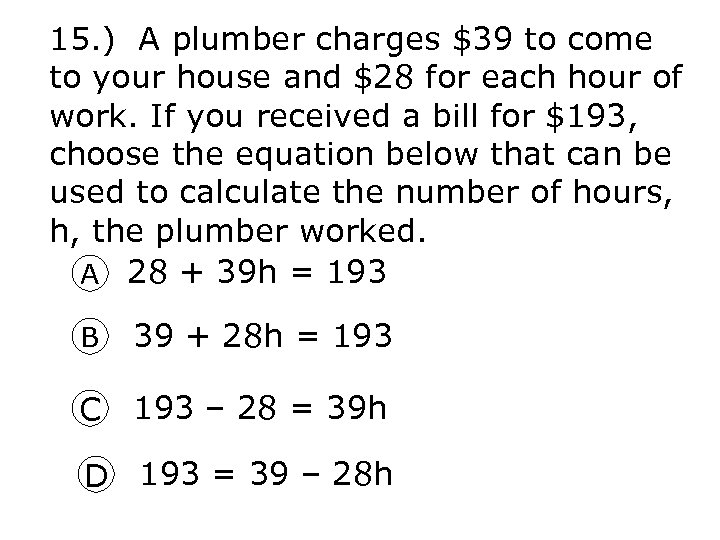15. ) A plumber charges \$39 to come to your house and \$28 for each hour of work. If you received a bill for \$193, choose the equation below that can be used to calculate the number of hours, h, the plumber worked. A 28 + 39 h = 193 B 39 + 28 h = 193 C 193 – 28 = 39 h D 193 = 39 – 28 h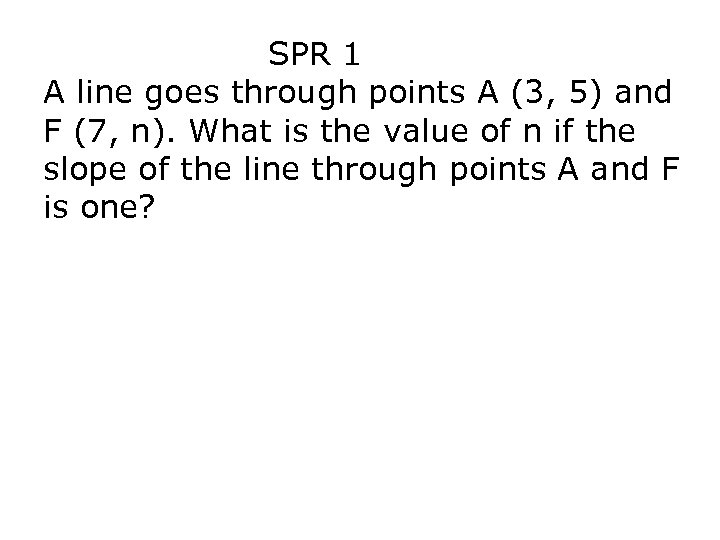SPR 1 A line goes through points A (3, 5) and F (7, n). What is the value of n if the slope of the line through points A and F is one?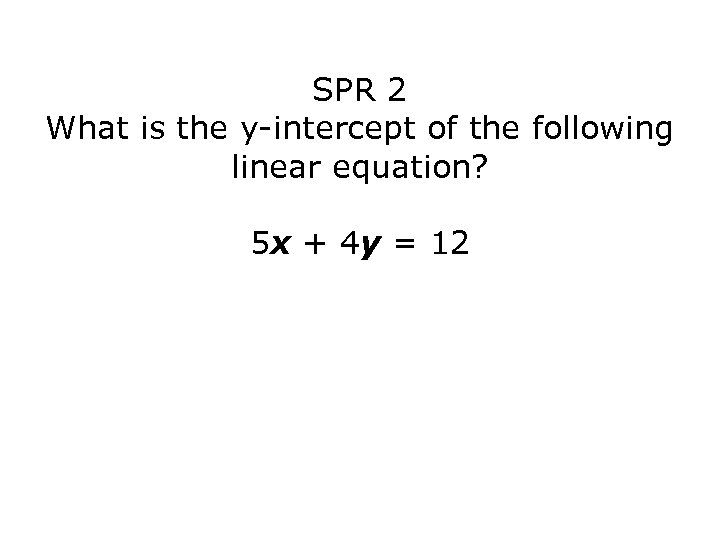SPR 2 What is the y-intercept of the following linear equation? 5 x + 4 y = 12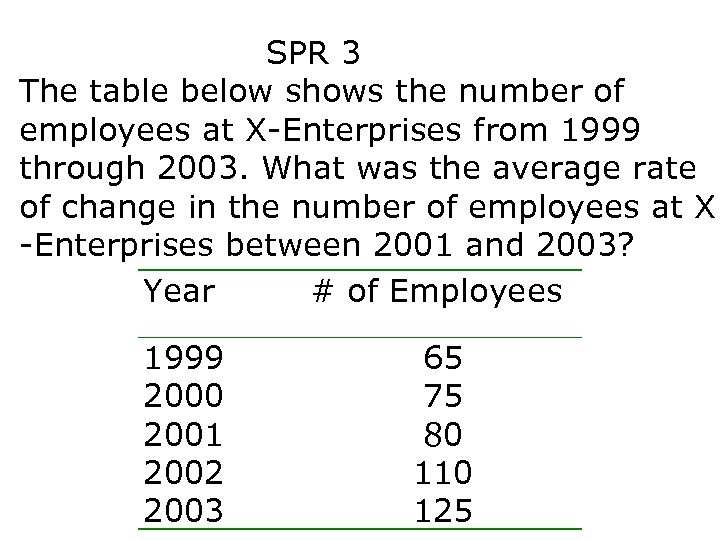SPR 3 The table below shows the number of employees at X-Enterprises from 1999 through 2003. What was the average rate of change in the number of employees at X -Enterprises between 2001 and 2003? Year # of Employees 1999 2000 2001 2002 2003 65 75 80 110 125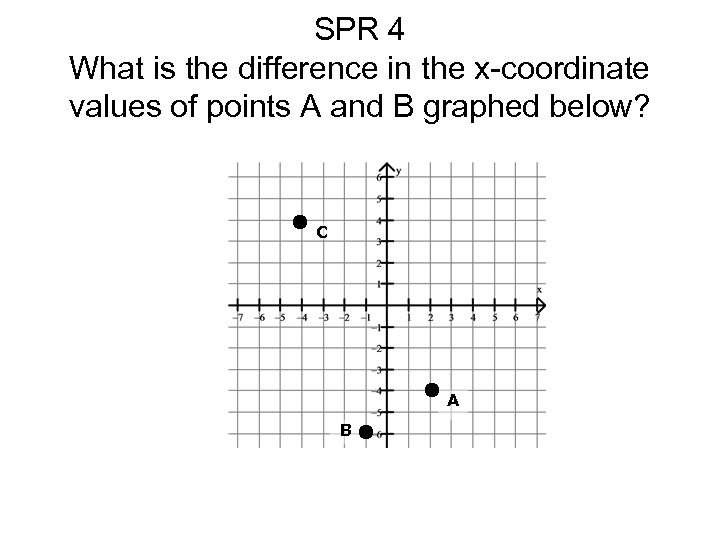SPR 4 What is the difference in the x-coordinate values of points A and B graphed below? C A B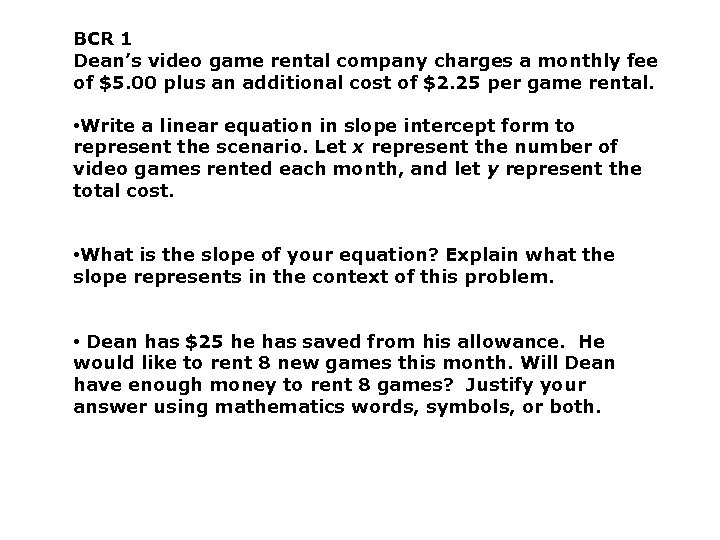BCR 1 Dean’s video game rental company charges a monthly fee of \$5. 00 plus an additional cost of \$2. 25 per game rental. • Write a linear equation in slope intercept form to represent the scenario. Let x represent the number of video games rented each month, and let y represent the total cost. • What is the slope of your equation? Explain what the slope represents in the context of this problem. • Dean has \$25 he has saved from his allowance. He would like to rent 8 new games this month. Will Dean have enough money to rent 8 games? Justify your answer using mathematics words, symbols, or both.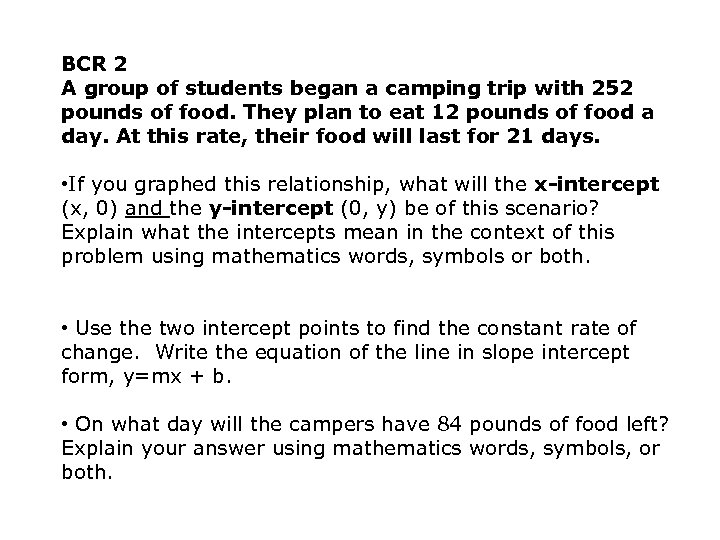BCR 2 A group of students began a camping trip with 252 pounds of food. They plan to eat 12 pounds of food a day. At this rate, their food will last for 21 days. • If you graphed this relationship, what will the x-intercept (x, 0) and the y-intercept (0, y) be of this scenario? Explain what the intercepts mean in the context of this problem using mathematics words, symbols or both. • Use the two intercept points to find the constant rate of change. Write the equation of the line in slope intercept form, y=mx + b. • On what day will the campers have 84 pounds of food left? Explain your answer using mathematics words, symbols, or both.